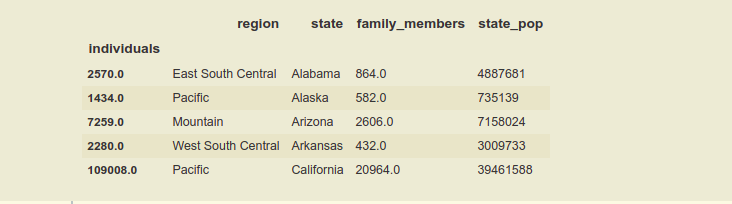# Reverting from multiindex to single index dataframe in Pandas

• Difficulty Level : Medium
• Last Updated : 21 Apr, 2021

In this article, we will be reverting from a multi-index to a single index in the pandas dataframe. Sometimes, when we are doing exploratory data analysis or data manipulation, we have to make the multi-indexing in dataframe, for extracting meaningful insights or to use the columns efficiently and easily. After doing the data manipulation, if we want to change our dataframe from multi-index to single index, so as it looks better, and it is recommended to change the dataframe into a single index.

The index is like an address, that’s how any data point across the dataframe or series can be accessed. Rows and columns both have indexes, rows indices are called index and for columns.

In this article, we are going to use homelessness.csv file for dataframe and apply methods to it.

## Python3

 `# importing pandas library as alias pd.``import` `pandas as pd`` ` `# using pandas read_csv().``df ``=` `pd.read_csv(``'homelessness.csv'``)``df.head()`

Output:As we can see that this dataframe, has no index. So, we create an index with multi-indexing by using the pandas set_index(), passing the name of the column names as the list.

## Python3

 `# making the 'region' and 'state' column as index.``df_mi ``=` `df.set_index([``'region'` `, ``'state'` `, ``'individuals'``])`` ` `print``(df_mi.head())`

Output:Now, the dataframe has Hierarchical Indexing or multi-indexing.  To revert the index of the dataframe from multi-index to a single index using the Pandas inbuilt function reset_index().

Syntax: DataFrame.reset_index(level=None, drop=False, inplace=False, col_level=0, col_fill=”)

Returns: (Data Frame or None) DataFrame with the new index or None if inplace=True.

Reverting the Multi-index using the above way i.e; using reset_index() we can follow as:

1. By using the level of the index.
2. By using the name of the index.

### By using the level of the index

Passing the values to the function by using the level keyword, which accepts the list of the levels, which we want to revert from the index position. As we know the multi-indexes form a hierarchy of indexes, that’s why these are also known as hierarchical indexes. In this Dataframe, ‘region’ is the level(0) index or the main index and the ‘state’ is the level(1) index and ‘individuals’ is the level(2) index. Converting the Dataframe into a single index only, by keeping the ‘state’ index and reverting ‘region’ and ‘individuals’ index.

## Python3

 `# using the reset_index(), reverting the``# level 0 and level 2 indexes.``df_si_level ``=` `df_mi.reset_index( level ``=` `[``0` `, ``2``] )`` ` `print``(df_si_level.head())`

#### Output:### By using the name of the index

In this method simply passing the name of the indexes in a list to the reset_index(). Converting the dataframe to a single index only, by keeping the ‘individuals‘ index and reverting the ‘region‘ and ‘state‘ index, just bypassing their names in the list.

## Python3

 `# using the reset_index(), reverting ``# the 'region' and 'state' indexes.``df_si_name ``=` `df_mi.reset_index([ ``'region'` `, ``'state'` `])`` ` `print``(df_si_name.head())`

#### Output:Note: If we wish to make the Dataframe, index-free, or we don’t want to make any column as an index, so in that case, we can pass the name of all the indexes or levels in the reset_index(), to make the Dataframe index free.

My Personal Notes arrow_drop_up